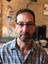Search
•Jack Orenstein

# Building new commands

Grep is very useful, and you can call it from marcel, e.g.

`grep "def set_env *.py`

Running this in marcel's marcel/op directory yields:

```assign.py:    def set_env(self, env):
ifbase.py:    def set_env(self, env):
map.py:    def set_env(self, env):
red.py:    def set_env(self, env):
runpipeline.py:    def set_env(self, env):
select.py:    def set_env(self, env):
sort.py:    def set_env(self, env):
squish.py:    def set_env(self, env):
sudo.py:    def set_env(self, env):
window.py:    def set_env(self, env):```

This output is not structured, so it is difficult to process further. It would be nice if there were a marcel version of grep that produced structured output, e.g.

```(assign.py, '    def set_env(self, env):')
(ifbase.py, '    def set_env(self, env):')
(map.py, '    def set_env(self, env):')
(red.py, '    def set_env(self, env):')
(runpipeline.py, '    def set_env(self, env):')
(select.py, '    def set_env(self, env):')
(sort.py, '    def set_env(self, env):')
(squish.py, '    def set_env(self, env):')
(sudo.py, '    def set_env(self, env):')
(window.py, '    def set_env(self, env):')```

A simple version of grep can be built in marcel itself, using parameterized pipelines:

```grem = [pattern, files: read --label (files) | \
select (file, line: pattern in line)]

```

This new (pseudo-) operator is named grem. It has two parameters: pattern, a string to search for; and files, a file name or glob. (Pipeline parameters don't yet support *, so you are limited to exactly one pattern and one glob when using grem.) The read operator reads the contents of each file, and the --label flag includes a label with each line, the File containing that line. The select operator is used to keep only those (file, line) pairs in which the line contains the given pattern.

So now you can write grem "def set_env" *.py and get the structured result shown above.

If you want grem to be available in all marcel sessions, you can define it in your config file (~/.marcel.py), assigning it to the RUN_ON_STARTUP variable, e.g.

```RUN_ON_STARTUP = '''
grem = [pattern, files: read -l (files) | select (f, l: pattern in l)]
'''```

(You can add more marcel commands to RUN_ON_STARTUP.)